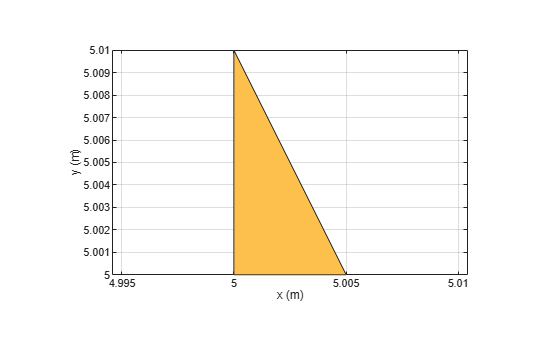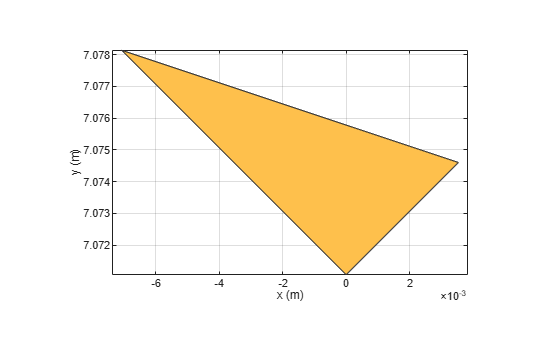# antenna.Triangle

Create triangle on X-Y plane

Since R2023a

## Description

Use the `antenna.Triangle` object to create a triangular shape in the X-Y plane with one vertex at the origin. You can use `antenna.Triangle` shape to create single-layer or multi-layered antenna using the `pcbStack`.

## Creation

### Syntax

``tri=antenna.Triangle``
``tri=antenna.Triangle(Name=Value)``

### Description

example

````tri=antenna.Triangle` creates a triangular shape in the X-Y plane with one vertex at the origin.```

example

````tri=antenna.Triangle(Name=Value)` creates a triangle, with additional Properties specified by one or more name–value arguments. `Name` is the property name and `Value` is the corresponding value. You can specify several name-value arguments in any order as `Name1`= `Value1`, `...`, `NameN`=`ValueN`. Properties not specified retain their default values.For example, `tri = antenna.Triangle(InputType="SSS",Side=0.01)` creates a triangle using side-side-side congruence with 10mm side length.```

## Properties

expand all

Name of the triangular shape, specified as a string.

Example: `"TriangleA"`

Data Types: `string`

Congruency condition for the triangle specified as a string. Default condition is `"SSS"`(side-side-side). Other supported conditions are `"SAS"`(side-angle-side) and `"ASA"`(angle-side-angle).

Example: `"SAS"`

Data Types: `string`

Coordinates of the start point to draw the triangle, specified as a 1-by2 vector in meter. It is the intersection point between the base side and the left side separated with an angle.

Example: `[5 5]`

Data Types: `double`

Length of the triangle side, specified as a positive scalar in meter. The default side length is 5 mm and `InputType` is `"SSS"`. When `InputType` is

• `"SSS"`, `Side` accepts a positive 1-by-3 vector for unequal side lengths or a positive scalar for equal side lengths.

• `"SAS"`, `Side` accepts a positive 1-by-2 vector for unequal side lengths or a positive scalar for equal side lengths.

• `"ASA"`, `Side` accepts a positive scalar.

Example: `1e-3`

Example: `[5e-3 10e-3]`

Example: `[3e-3 4e-3 5e-3]`

Data Types: `double`

Adjacent side angle of the triangle in degrees, specified as a positive scalar or a positive 1-by-2 vector. This property is activated only when the `InputType` is either `"SAS"` or `"ASA"`. It is the angle between the base side and left adjacent side (sides which share the `StartVertex`) for `"SAS"` congruence and angle between base side and other two adjacent sides for `"ASA"` congruence. This property accepts

• A positive scalar value for `"SAS"` congruence.

• A positive scalar value for equal angles and a positive 1-by-2 vector for unequal angles in `"ASA"` congruence.

Example: `45`

Example: `[20 30]`

Data Types: `double`

## Object Functions

 `add` Add data to polar plot `subtract` Boolean subtraction operation on two shapes `intersect` Boolean intersection operation on two shapes `plus` Shape1 + Shape2 `minus` Shape1 - Shape2 `and` Shape1 & Shape2 `area` Calculate area of shape in square meters `show` Display antenna, array structures or shapes `plot` Plot boundary of shape `mesh` Mesh properties of metal, dielectric antenna, or array structure `rotate` Rotate shape about axis and angle `rotateX` Rotate shape about x-axis and angle `rotateY` Rotate shape about y-axis and angle `rotateZ` Rotate shape about z-axis and angle `translate` Move shape to new location `scale` Change the size of the shape by a fixed amount `mirrorX` Mirror shape along X-axis `mirrorY` Mirror shape along Y-axis `removeHoles` Remove holes from shape `removeSlivers` Remove sliver outliers from boundary of shape

## Examples

collapse all

This example shows how to create a traingular shape when two side dimensions and angle between them is known and rotate it by 45-degrees about the z-axis.

Create Traingular Shape

Create a right angled triangular shape with length of one side as 5 mm and the length of other side as 10 mm, and starting vertex [5 5].

Display the created shape.

```t = antenna.Triangle(Name="Right_Angled_Triangle",InputType="SAS",... Side=[5e-3 10e-3],Angle=90,StartVertex=[5 5]); show(t)````rotateZ(t,45)`